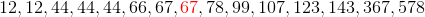SAT II Math I : Data Analysis and Statistics

Example Questions

Example Question #234 : Data Properties

Find the median of the set: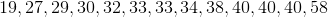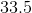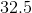Explanation:

The median is the middle number of the set, when it is listed in order from smallest to largest or vice versa. In this case we have an odd amount of numbers so we just count from each side until we find the number in the middle.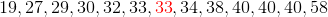This gives us a final answer of 33 for the median.

Example Question #235 : Data Properties

FInd the median of the set: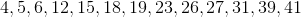Explanation:

The median is the middle number of the set, when it is listed in order from smallest to largest or vice versa. In this case we have an odd amount of numbers so we just count from each side until we find the number in the middle.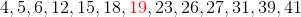This gives us a final answer of 19 for the median.

Example Question #31 : Median

Find the median of the set: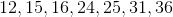Explanation:

The median is the middle number of the set, when it is listed in order from smallest to largest or vice versa. In this case we have an odd amount of numbers so we just count from each side until we find the number in the middle.This gives us a final answer of 24 for the median.

Example Question #32 : Median

Find the median of the set: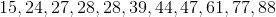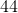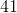Explanation:

The median is the middle number of the set, when it is listed in order from smallest to largest or vice versa. In this case we have an odd amount of numbers so we just count from each side until we find the number in the middle.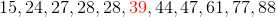This gives us a final answer of 39 for the median.

Example Question #33 : Median

Find the median of the set: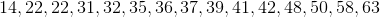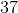Explanation:

The median is the middle number of the set, when it is listed in order from smallest to largest or vice versa. In this case we have an odd amount of numbers so we just count from each side until we find the number in the middle.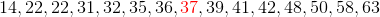This gives us a final answer of 37 for the median.

Example Question #34 : Median

Find the median of the following number set: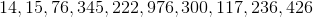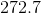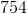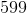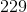Explanation:

Find the median of the following number set:Recall that the median is the middle value of a number set when it is arranged in ascending order. So we must begin by arranging the set in ascending order.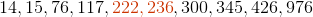In this case, because we have an even number of terms, we do not have a single middle number. This means we need to take the average of the middle two terms to find our median.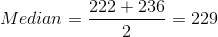Example Question #35 : Median

Find the median: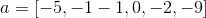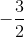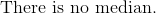Explanation:

First regroup all numbers in chronological order.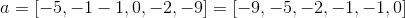Since there is an even amount of numbers in the dataset, 6 numbers total, the median will be the average of the third and fourth numbers.

Find the mean of the two numbers.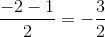Example Question #241 : Data Properties

Find the median of the following data set: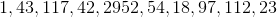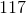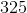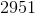Explanation:

Find the median of the following data set: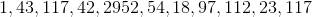To find the median, we should first put our numbers in increasing order: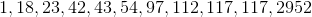Next, the median will simply be the number in the middle.

We have 11 terms, so our median will be the 6th term in the sequence: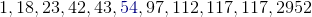So 54 is our median

Example Question #242 : Data Properties

Find the median of this data set: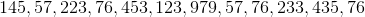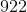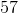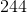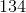Explanation:

Find the median of this data set:To begin, let's put our numbers in increasing order: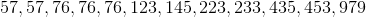Next, find the median by finding the number in the middle of  the data set. If there are two numbers in the middle, then find their average: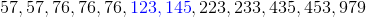So, the median will be: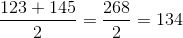Example Question #243 : Data Properties

Find the median of the following data set: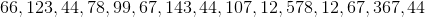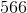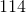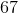Explanation:

Find the median of the following data set:Let's begin by putting our data in increasing order: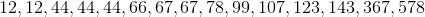Now, the median should be pretty straightforward to find.

The median will simply be the number in the middle of all the terms.

In this case, it is the number in red below